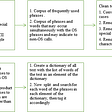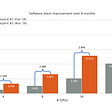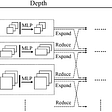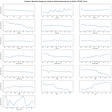# Why “Linear Algebra” is cool ??

Linear Algebra is to machine learning as flour to bakery: Every machine learning model is based on linear algebra, as every cake is base on flour (But ML model is not about only Linear algebra, they need calculus, probability, and optimization too).

## WHY LINEAR ALGEBRA ??

Linear algebra via the use of matrices and vectors, allows us to perform a large number of calculations in a more efficient way while using simpler code with the help of linear algebra library (such as NumPy). Linear Algebra relates variables(Dependent Variables, Independent Variables, and Target Variables) by a mathematical relationship.

## Vectors

Vectors are ordered finite lists of numbers. It is a type of mathematical object in which we can perform mathematical operations to obtain another object of the same kind (vector ). Vector is a special case of matrix, that has only 1 column, i.e., (m x 1) matrix, also known as a column vector. We can think of a matrix as a group of column vectors or row vectors (1 x n matrix).

We can use vectors to represent attributes of entities: age, balance, sex, test scores, etc. By convention, we denote vectors with a lowercase letter.

Here, x is a vector of 3 dimensional having 1 element on each, or we can say it as a 3 x 1 matrix. Thus, it is an element of the set R^(3 x 1) or , since it is a vector. In python, vectors are 0-indexed i.e the first entry in a vector is 0th element.

This snippet shows how can we have fun(perform different operations) with vectors. such as a+b results summation of a and b vectors. And here “*” (multiplication operator) results in a product of scalar with a vector.

We can recall Linear combination as a resultant combination of mathematical operation “addition” and “multiplication” with vector. i.e, ax+by=a[x1,x2]+b[y1,y2]=[[ax1+ax2 ] [by1+by2]] , where x and y are vector with corresponding elements.

## Linear Dependence and Linear Independence

A set of vectors is linearly dependent if at least one vector can be obtained as a linear combination of other vectors in a set. In other words, A set of vectors {v1,v2,...,vk} is linearly dependent if there exist numbers x1,x2,x3,…xk, not all equal to 0, in equation x1.v1+x2.v2+···+xk.vk=0 .

A set of vectors is said to be linearly independent if none vector can be obtained as a linear combination of other vectors. In other words, a set of vectors {v1,v2,…,vk} is linearly independent if the vector equation x1.v1+x2.v2+···+xk.vk=0 has the one trivial solution i.e., x1=x2=x3=….=xk

Linearly dependent vectors contain redundant information, whereas linearly independent vectors do not.

## Matrices

Matrices are as fundamental as vectors in Machine learning. A matrix is a rectangular array of numbers. Those numbers contained in square brackets. It is a 2-dimensional array, made up of rows and columns.

With vectors, we can represent single variables as sets of numbers or objects. With a matrix, we can represent sets of variables or vectors.

With matrix-matrix multiplication to work, the number of columns in the first matrix “A” has to be equal to the number of rows in the second matrix “x”. The resultant matrix will be of the shape (rows of matrix “A” and column of matrix “x”).

## Matrix Transpose

Taking the transpose of a matrix means that the rows of that matrix become the columns. Thus, the first row of matrix A becomes the first column of A^T (the transpose of matrix A), and the second row of matrix A becomes the second column of A^T. Thus, if matrix A is an mxn matrix, then its transpose, or A^T, is an nxm matrix.

Consider a matrix A∈R³*². The transpose of A is denoted as (A^T)∈R²*³.

In other words, we get the (A^T) by switching the columns by the rows of A.

## References

Go through Essence of linear algebra for 3blue 1 brown https://www.youtube.com/playlist?list=PLZHQObOWTQDPD3MizzM2xVFitgF8hE_ab

Introduction to Applied Linear Algebra -Vectors, Matrices, and Least Squares http://vmls-book.stanford.edu/

--

--

--

## More from Analytics Vidhya

Analytics Vidhya is a community of Analytics and Data Science professionals. We are building the next-gen data science ecosystem https://www.analyticsvidhya.com

## Labelling unstructured text data in Python## NLP with R part 1: Topic Modeling to identify topics in restaurant reviews## Lessons for Improving Training Performance — Part 1## Knowledge Distillation in a Deep Neural Network## Understanding a Black-Box## Basic FAQ Chatbot using Machine Learning## Flow-Forecast: A time series forecasting library built in PyTorch## Apply computer vision on the questionaire image to detect ticked checkboxes## Abu Qais

The price of “anything” is the amount of “time”, U xchange for it. Education | Technology | Data Science | Statistics | History

## Creating Neuro-Symbolic Sudoku Solver## The Missing Library in your Machine Learning Workflow## Predicting Parkinsonian Symptom Severity from Voice Recordings